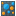#ANOVA Matrix (RapidMiner Studio Core)

## Synopsis

This operator performs an ANOVA significance test for all numerical attributes based on the groups defined by all the nominal attributes. ANOVA is a general technique that can be used to test the hypothesis that the means among two or more groups are equal, under the assumption that the sampled populations are normally distributed.

## Description

The ANalysis Of VAriance (ANOVA) is a statistical model in which the observed variance in a particular variable is partitioned into components attributable to different sources of variation. In its simplest form, ANOVA provides a statistical test of whether or not the means of several groups are all equal, and therefore generalizes a t-test to more than two groups. Doing multiple two-sample t-tests would result in an increased chance of committing a type I error. For this reason, ANOVA is useful in comparing two, three, or more means. 'False positive' or Type I error is defined as the probability that a decision to reject the null hypothesis will be made when it is in fact true and should not have been rejected. In the typical application of ANOVA, the null hypothesis is that all groups are simply random samples of the same population. This implies that all treatments have the same effect (perhaps none). Rejecting the null hypothesis implies that different treatments result in altered effects.

## Differentiation

###Grouped ANOVA

The Grouped ANOVA operator performs ANOVA significance test for the user-specified anova attribute (numerical) based on the groups defined by user-specified attribute (nominal).

## Input

•example set (Data Table)

This input port expects an ExampleSet. It is the output of the Retrieve operator in the attached Example Process. The output of other operators can also be used as input. The ExampleSet should have both nominal and numerical attributes because this operator performs an ANOVA significance test for all numerical attributes based on the groups defined by all the nominal attributes.

## Output

•example set (Data Table)

The ExampleSet that was given as input is passed without changing to the output through this port. This is usually used to reuse the same ExampleSet in further operators or to view the ExampleSet in the Results Workspace.

•anova (ANOVA Matrix)

The ANOVA significance test for all numerical attributes is performed based on the groups defined by all the nominal attributes. The resultant ANOVA matrix is returned from this port.

## Parameters

• significance_levelThis parameter specifies the significance level for the ANOVA calculation. Range: real
• only_distinctThis parameter indicates if only rows with distinct values of the aggregation attribute should be used for the calculation of the aggregation function. Range: boolean

## Tutorial Processes

### ANOVA matrix of the Golf data set

The 'Golf' data set is loaded using the Retrieve operator. A breakpoint is inserted here so that you can view the ExampleSet. You can see that the ExampleSet has both nominal and numerical attributes. The ANOVA Matrix operator is applied on this ExampleSet. This operator performs an ANOVA significance test for all numerical attributes based on the groups defined by all the nominal attributes. The resultant ANOVA matrix can be viewed in the Results Workspace.